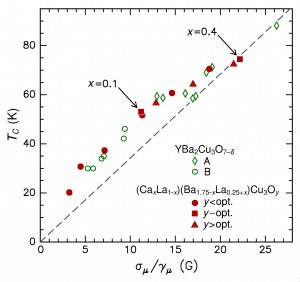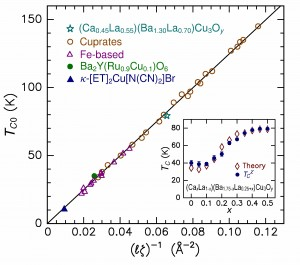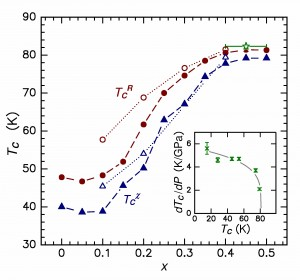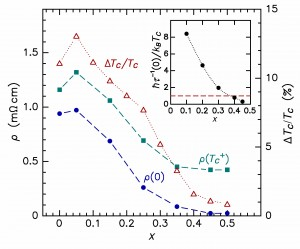# Charge Compensation and Optimal Stoichiometry in Superconducting (CaxLa1–x)(Ba1.75–xLa0.25+x)Cu3Oy

Charge Compensation and Optimal Stoichiometry in Superconducting (CaxLa1–x)(Ba1.75–xLa0.25+x)Cu3Oy, D. R. Harshman and A. T. Fiory [arXiv]
The superconductive and magnetic properties of charge-compensated (CaxLa1–x)(Ba1.75–xLa0.25+x)Cu3Oy (normally denoted as CLBLCO) are considered through quantitative examination of data for electrical resistivity, magnetic susceptibility, transition width, muon-spin rotation, x-ray absorption, and crystal structure. A derivative of LaBa2Cu3Oy, cation doping of this unique tetragonal cuprate is constrained by compensating La substitution for Ba with Ca substitution for La, where for 0 ≤ x ≤ 0.5 local maxima in TC occur for y near 7.15. It is shown that optimum superconductivity occurs for 0.4 ≤ x ≤ 0.5, that the superconductivity and magnetism observed are nonsymbiotic phenomena, and that charge-compensated doping leaves the carrier density in the cuprate planes nearly invariant with x, implying that only a small fraction of superconducting condensate resides therein. Applying a model of electronic interactions between physically separated charges in adjacent layers, the mean in-plane spacing between interacting charges, ℓ = 7.1206 Å, and the distance between interacting layers, ζ = 2.1297 Å, are determined for x = 0.45. The theoretical optimal TC0 ∝ ℓ–1ζ–1 of 82.3 K is in excellent agreement with experiment (≈ 80.5 K), bringing the number of compounds for which TC0 is accurately predicted to 37 from six different superconductor families (overall accuracy of ±1.35 K).Transition temperature measured by μ+SR plotted against the μ+SR Gaussian relaxation parameter divided by the muon gyromagnetic ratio, σμ/γμ, for polycryst. samples of CLBLCO with depleted oxygen at x = 0.4 (filled circles), excess oxygen at x = 0.4 (filled triangles), and optimum oxygen at x = 0.1 and x = 0.4 (filled squares). Similarly obtained μ+SR data for ceramic samples of YBa2Cu3O7–δ for various δ are presented for comparison. The dashed line represents a non-existent proportional behavior.Experimental TC0 versus (ℓζ)–1, where ℓ is intra-layer mean spacing of interacting charges and ζ is inter-layer interaction distance, for CLBLCO (x = 0.45, star symbol), compared to other cuprates (open circles), Fe-based pnictides and chalcogenides (open triangles), a ruthenate (filled circle) and an organic (filled triangle). The solid line through the data points represents theory. Inset: transition temperatures of CLBLCO (filled symbols) and pair-breaking theory (open symbols) as function of doping x.Transition temperatures TC for CLBLCO, maximized by oxygen content y, extracted from resistivity (filled circles after Ref. 1, open circles after Ref. 4) and magnetic susceptibility (filled triangles after Ref. 1, open triangles after Ref. 2) as function of x. Star symbol denotes theoretical TC0 averaged over x = 0.4 – 0.5. Inset represents dTC/dP versus TC after Ref. 2; curve denotes trend.Resistivity measurements ρ(TC+) and ρ(0), left scale, and the percentage transition widths ΔTC/TC, right scale, plotted as functions of x for (CaxLa1–x)(Ba1.75–xLa0.25+x)Cu3Oy, with y set to maximize TC (after Ref. 1). Inset shows ħt–1(0)/kBTC plotted against doping x. Horizontal dashed line denotes unity.

D. R. Harshman and A. T. Fiory, Phys. Rev. B 86, 144533 (2012).